I. INTRODUCTORY PROBLEMS

1. Find y the following:

(i)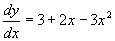(ii)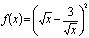(iii)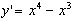(iv)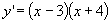(v)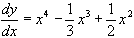(vi)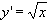(vii)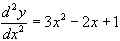(viii)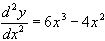(ix)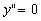2. In each of the following, find F(x) given that F'(x) = f(x):

(a)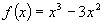(b)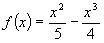(c)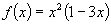(d)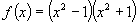(e)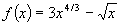(f)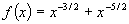(g)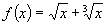3. Find f(x) given that f'(x) = 2x-2 and f(1) = 4. [ans: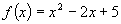]

1. Find f(x) given that f'(x) =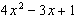and f'(1) = 3.
2. At all points on a certain curve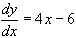. The point (2, 4) belongs to the curve. Find the equation of the curve. [ans: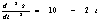]

6. Find the equation of the curve given that the gradient at any point P(x, y) is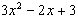and that the point (3,3) belongs to the curve.

7. For the function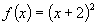find the rule that defines the function F, where F'(x) = f(x) and F(1) = 4.

8. Find the equation of a curve given that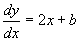at any point P and that when x=3,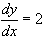and y = -3.

9. Find the rule that defines a function f, given that f'(x) =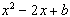for all x, and f'(0) = 1 and f(0) = 2.

10. A curve contains the point (0,4) and its gradient is (x-1)(x+2) at any point on the curve. Find the equation of the curve.

11. At all points on a certain curve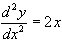. The point (3,6) belongs to the curve and its tangent at this point is inclined at 45o to the X-axis. Find the equation of the curve.

12. Find the primitives of

(a)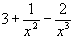(b)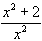(c)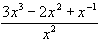(d)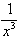.

13. In each of the following, find f(x) given that:

(a)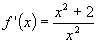(b)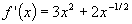(c)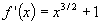(d)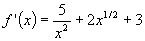14. Given that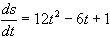, find s in terms of t given that s=4 when t=1.

15. Given that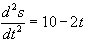, t>0 and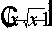and s=0 when t=0, find

(a) t when(b) s when.

[ans: (a) 12 (b) 432]

16. Given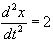for all t > 0 and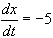and x = 4 when t = 0, find t when

(a)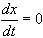(b) x = 0.

[ans: (a) 5/2 (b) 1 or 4]